February 25, 2020

## Key parts of computational thinking

Decomposition
breaking down a complex problem or system into smaller parts
Pattern Recognition
looking for similarities among and within problems
Abstraction
focusing on the important parts only, ignoring irrelevant detail
Algorithms
developing a step-by-step solution to the problem

## We can draw stars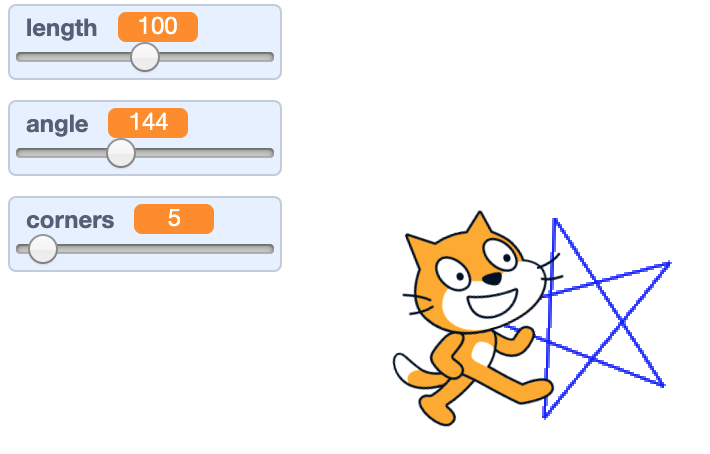## With a code like this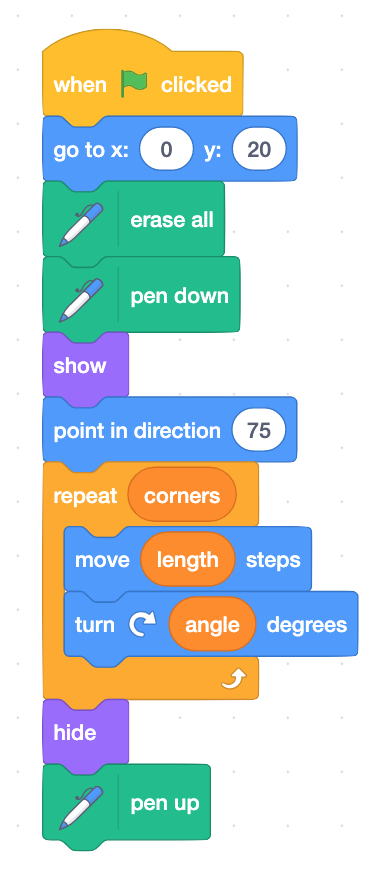## We can also draw circles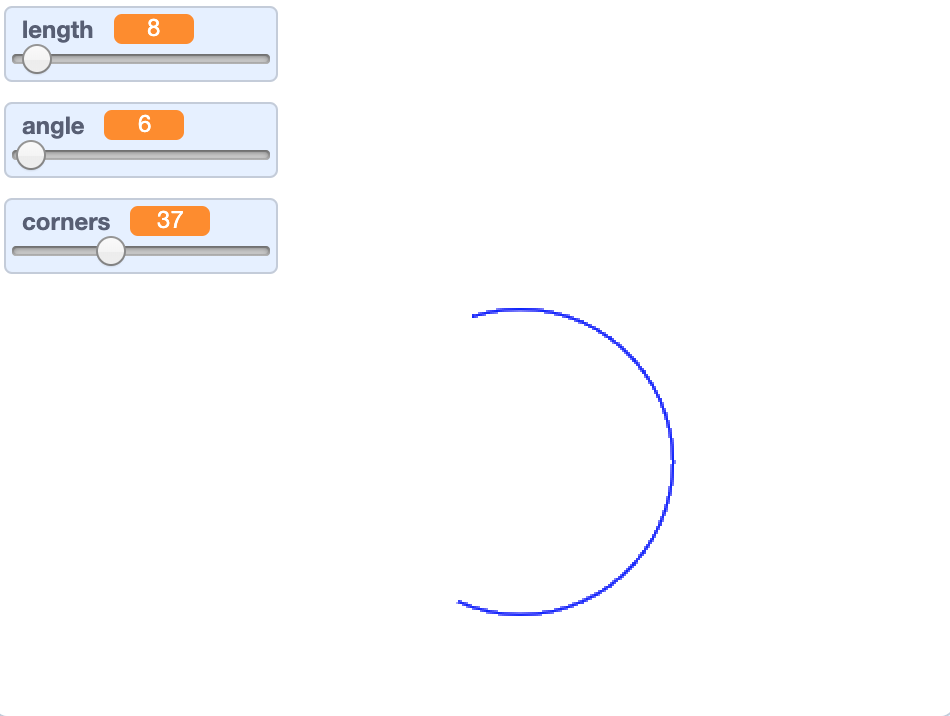## and draw something like the moon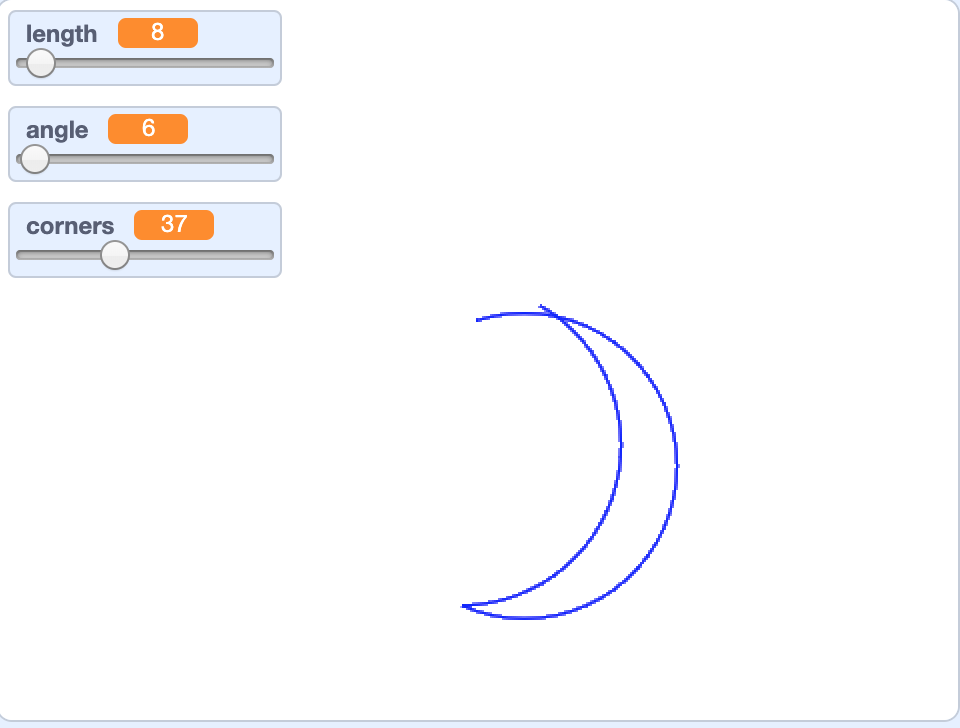## Now we will do the same in R

You can install TurtleGraphics in R with this command

install.packages("TurtleGraphics")

Then you load into the session using

library(TurtleGraphics)

Now you start a new “terrarium” with

turtle_init()

## Turtle lives in a terrarium

Set Up a new Terrarium

turtle_init(width = 100, height = 100, mode = "error")
width
numeric; plot width.
height
numeric; plot height.
mode
character string; one of “error”, “clip”, or “cycle”.
We will explore these options later

## The turtle can move

Move the Turtle Forward or Backward
turtle_move(10) move (10) steps
Turn (Rotate) the Turtle
turtle_right(15) turn cw (15) degrees
turtle_left(15) turn ccw (15) degrees
Set the Turtle’s Position and Direction
turtle_goto(0,0) go to x:(0) y:(0)
turtle_setangle(90) point in direction (90 v)
turtle_setpos(0,0) Move without drawing

## She can draw and hide

Pen commands
turtle_up() pen up
turtle_down() pen down
Display Options
turtle_col(0) set pen color to (0)
turtle_lwd(1) set pen size to (1)
turtle_lty(1) Change line type
Show or Hide the Turtle
turtle_hide() hide
turtle_show() show

## Where is the Turtle?

Get the Turtle’s Current Position and Direction
turtle_getangle() (direction)

turtle_getpos()

• (x position)
• (y position)

## “Turbo” Mode

Evaluate a Larger Portion of Turtle Drawing Code

• turtle_do({code here})

## By default the terrarium size is 100,100

turtle_init()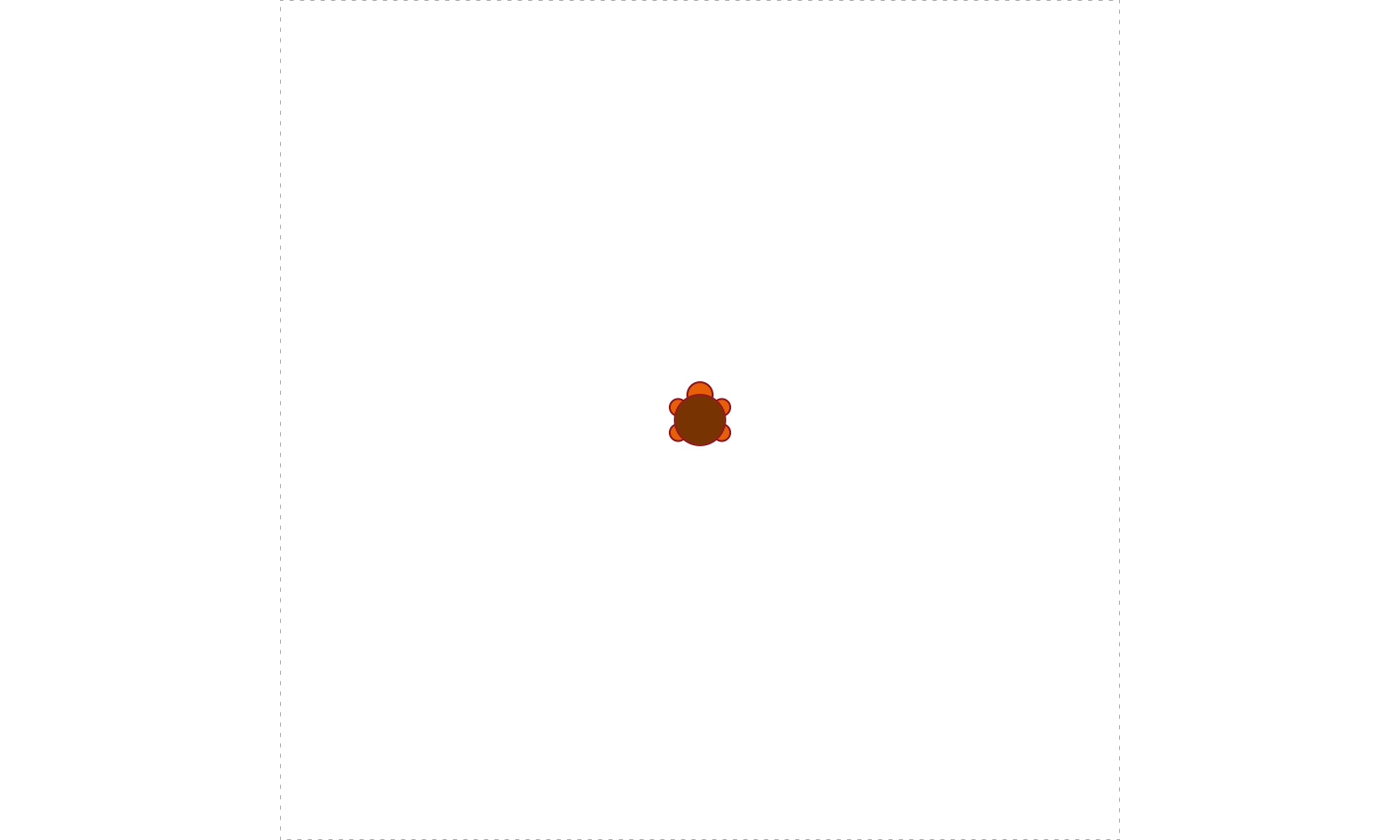## In R, the turtle is not at (0,0)

turtle_getangle()
## angle
##     0
turtle_getpos()
##  x  y
## 50 50

So we have to adapt our code (how?)

## Terrarium has borders

turtle_move(80)
## Error in .turtle_draw_error(distance, curX, curY, curAng, curGp, curDraw, : The Turtle escaped from the terrarium. :-(

## We can relax the border control

turtle_init(mode = "clip")## Exercise

Now you should be able to draw stars and moons in R

Write the code to draw a star in R

## Decomposition

Separating a complex problem into smaller, more manageable parts

Here we separate a complex figure into many smaller parts

## Pattern recognition

Is this problem similar to another?

Are there parts of this problem that are similar to each other?

## Algorithms: loops

You already have seen a first algorithmic structure: loops

repeat (10)

which in R we write as

for(i in 1:10) {
...
}

## Algorithms

The second algorithmic structure you can use are

Sub-routines
A group of specific steps grouped together

In R we represent sub-routines with functions

## Functions

In Math and Informatics, a function is a “black box”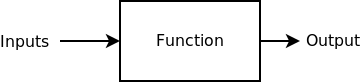A rule to transform the input elements into an output

• e.g. logarithm of a number, length of a vector

The same input should produce always the same output

Notice that there may be more than one input element

## Functions in R

In R functions are a type of data. We have

• numeric
• character
• logic
• factor
• formulas
• functions
• and others

To create a function we need to assign it to a variable

## Function syntax

newFunc <- function(input) {
commands
commands
return(output)
}

The keyword return can be omitted

In that case the output is the result of the last command

## Input values

• A function can get zero, one or several inputs
• They are mandatory, unless a default value is specified
• For example, if
my_func <- function(a, b=2) {return(a*b)}
my_func(3, 3)
##  9
my_func(3)
##  6

## What if the input is wrong?

my_func()
## Error in my_func(): argument "a" is missing, with no default
my_func(1,2,3,4)
## Error in my_func(1, 2, 3, 4): unused arguments (3, 4)

## Writing a function

Before writing the function we need to decide:

• the name of the function (it’s harder than it seems)
• which are the inputs
• what is the output

Then we can start writing the code

## What is computational thinking?

### from BBC website

Computers can be used to help us solve problems

Before a problem can be tackled, we need to understand the problem itself and the ways in which it could be solved

Computational thinking allows us to do this

## What is computational thinking?

### from BBC website

Computational thinking allows us to take a complex problem, understand what the problem is and develop possible solutions

We can then present these solutions in a way that a computer, a human, or both, can understand

## The four key techniques of computational thinking

• decomposition

• pattern recognition

• abstraction

• algorithm design

## Decomposition

Breaking down a complex problem or system into smaller, more manageable parts

## Pattern recognition

Looking for similarities among and within problems

## Abstraction

Focusing on the important information only, ignoring irrelevant detail

## Algorithm Design

Developing a step-by-step solution to the problem, or the rules to follow to solve the problem

## Each cornerstone is as important as the others

They are like legs on a table

if one leg is missing, the table will collapse

Correctly applying all four techniques will help when programming a computer.

## Computational thinking in practice

A complex problem is one that, at first glance, we don’t know how to solve easily.

Computational thinking involves taking that complex problem and breaking it down into a series of small, more manageable problems (decomposition). Each of these smaller problems can then be looked at individually, considering how similar problems have been solved previously (pattern recognition) and focusing only on the important details, while ignoring irrelevant information (abstraction). Next, simple steps or rules to solve each of the smaller problems can be designed (algorithms).

Finally, these simple steps or rules are used to program a computer to help solve the complex problem in the best way.

## It is not about computers

Computational thinking is about problem solving

Almost any problem can be solved using computational thinking

For example: Sports, Projects, Science

## Homework 3Using R Turtle Graphics, write programs to draw:

• A house
• A person
• A pentagon
• A polygon of N sides

Filename must be homework3.R.
Send it to andres.aravena+cmb@istanbul.edu.tr
Write your student number in the email’s Subject